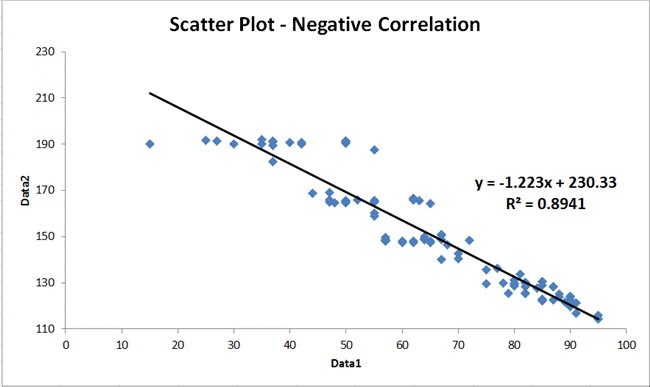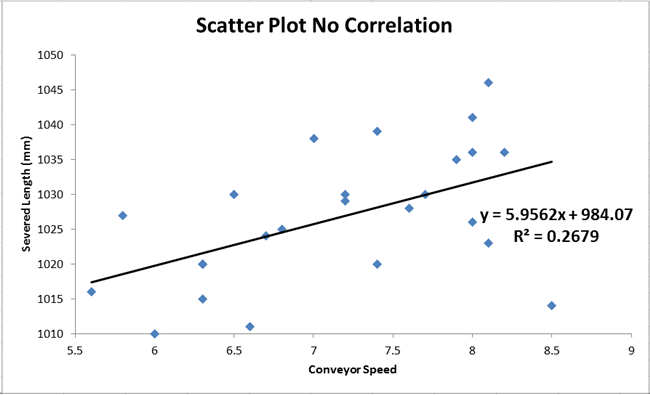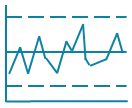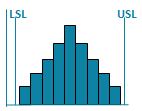# Scatter Plot Examples

Scatter plots are used to evaluate the correlation or cause-effect relationship (if any) between two variables.

## There are three primary types of scatter plots:

### Strong Positive CorrelationData points are clustered along a trend line
Upward slope (as one variable increases so does the other).
R² is greater than .80

### Strong Negative CorrelationData points are clustered along a trend line.
Downward slope (as one variable increases the other decreases.)
R² is greater than .80

### No CorrelationData points look like a shotgun blast
R² is less than .80### Calculating the Correlation of Determination

If R², the correlation of determination (square of the correlation coefficient), is greater than 0.8, then 80% of the variability in the data is accounted for by the equation. Most statistics books imply that this means that you have a strong correlation.

Scatter Plots can be made manually or in Excel. However, you have to find the right chart to get a trend line and Excel will not calculate the R² for you.

QI Macros Add-in for Excel can create a scatter plot in seconds and will calculate the slope and R² for you.

### QI Macros Can Also Create These Charts in Excel

Control ChartsHistogramsPareto ChartsBox PlotsFishbone Diagram### Why Choose QI Macros for Excel?### Affordable

• Only \$329 USD - less with quantity discounts
• No annual subscription fees
• Free technical support### Easy to Use

• Works right in Excel
• Create a chart in seconds
• Easy to customize & share charts### Proven and Trusted

• More than 100,000 users
• In More than 80 countries
• Five Star CNET Rating - Virus free